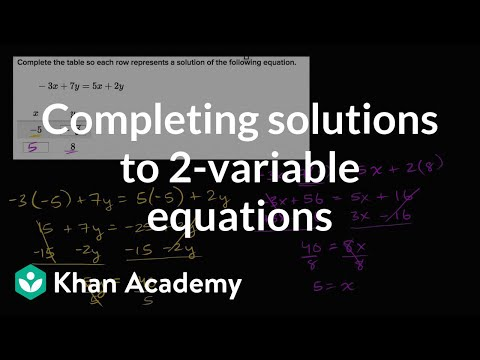Video

# Systems of equations with elimination: 4x - 2y = 5 and 2x - y = 2.5 (Full video)

Khan Academy

## Supporting grades: 8

Description: Sal demonstrates how to solve the system of equations 4x - 2y = 5 and 2x - y = 2.5 using the elimination method. We're told to solve and graph the solution for the system of equations right here. So let's multiply this equation, this second equation, by negative 2. So I'm going to multiply both sides of this equation by negative 2.

You must log inorsign upif you want to:*

*Teacher Advisor is 100% free.

### Other videos you might be interested in### Solving Systems of Equations by Substitution (Full video)

#### Student Achievement Partners